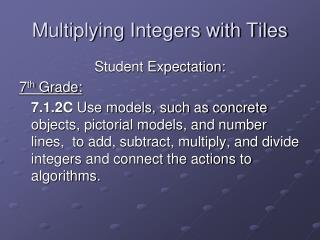DownloadDownload PresentationMultiplying Integers with Tiles

# Multiplying Integers with Tiles

Télécharger la présentation## Multiplying Integers with Tiles

- - - - - - - - - - - - - - - - - - - - - - - - - - - E N D - - - - - - - - - - - - - - - - - - - - - - - - - - -
##### Presentation Transcript

1. Multiplying Integers with Tiles Student Expectation: 7th Grade: 7.1.2C Use models, such as concrete objects, pictorial models, and number lines, to add, subtract, multiply, and divide integers and connect the actions to algorithms.

2. MULTIPLICATION • Red and yellow tiles can be used to model multiplication. • Remember that multiplication can be described as repeated addition. • So 2 x 3 = ? 2 groups of 3 tiles = 6 tiles The University of Texas at Dallas

3. MULTIPLICATION • 2 x -3 means 2 groups of -3 2 x -3 = -6 The University of Texas at Dallas

4. MULTIPLICATION • Since 2 x 3 = 6 and 3 x 2 = 6, does it make sense that -3 x 2 = -6 ? • -3 x 2 = -6 is true. • +2 x -3 = -6 and -3 x +2 = -6 belong to a fact family: +2 x -3 = -6 -3 x +2 = -6 -6 ÷ +2 = -3 -6 ÷-3 = +2 The University of Texas at Dallas

5. MULTIPLICATION • Use your tiles to model each multiplication problem. • +2 x +4 = ? ANSWER 2 groups of +4 +2 x +4 = +8 The University of Texas at Dallas

6. MULTIPLICATION • +3 x -3 = ? ANSWER 3 groups of -3 +3 x -3 = -9 The University of Texas at Dallas

7. MULTIPLICATION • -2 x +4 = ? ANSWER 4 groups of -2 -2 x +4 = -8 • Use the fact family for • -2 x +4 = ?  We can’t show -2 groups of +4 • +4 x -2 = ? we can show 4 groups of -2 The University of Texas at Dallas

8. MULTIPLICATION • +1, -1 are opposites the products are opposite • Since +2 and -2 are opposites of each other, • +2 x -3 and -2 x -3 have opposite products. +1 x +3 = +3 -1 x +3 = -3 The University of Texas at Dallas

9. MULTIPLICATION • To model -2 x -3 use 2 groups of the opposite of -3 -2 x -3 = +6 The University of Texas at Dallas

10. MULTIPLICATION • Use tiles to illustrate -3 x -4 =? ANSWER 3 groups of +4 (the opposite of -4) -3 x -4 = +12 The University of Texas at Dallas